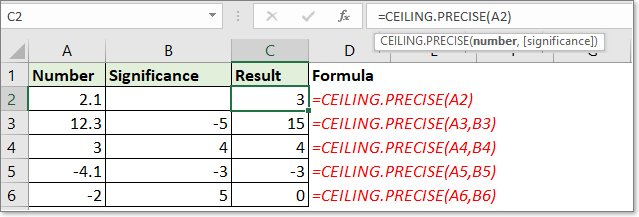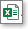## Excel CEILING.PRECISE function### Description

The CEILING.PRECISE function returns a number that is rounded up to the nearest integer or to the nearest multiple of specified significance. Differing with CEILING and CEILING.MATH functions, the CEILING.PRECISE function ignores the sign of the given number, and returns a number which is always rounded up.

### Syntax and arguments

Formula syntax

 =CEILING.PRECISE(number, [significance])

Arguments

 Number: Required. The number value you want to round. Significance: Optional. The multiple of significance which you use to round the number to. If this argument is omitted, the number is rounded up to the nearest multiple of 1.

Return Value

The CEILING.PRECISE function rounds number up to nearest integer or nearest multiple.

Notes:

1. The result of number is always rounded up whatever the given number is positive or negative.

2. If the significance or number is zero, zero will be returned.

3. Positive number is rounded away from zero, but negative number is rounded toward zero.

4. The sign of the [significance] argument is ignored, the results are same for both positive and negative [significance] argument. I.e. =CEILING.PRECISE(2,3) returns 3, and =CEILING.PRECISE(2,-3) also returns 3.

5. If the (number) argument is negative, and the [significance] argument is smaller than the (number) argument, it returns 0. I.e.=CEILING.PRECISE(-3,-4) returns 0, but =CEILING.PRECISE(-3,-2) returns -2.

### Usage and Examples

Example 1 – Work for positive number

 Number Significance Description Formula Result 2.1 Round 2.1 up to nearest multiple of 1 =CEILING.PRECISE(2.1) 3 3.3 3 Round 3.3 up to nearest multiple of 3 =CEILING.PRECISE(3.3,3) 6 4 -5 Round 4 up to nearest multiple of -5 =CEILING.PRECISE(4,-5) 5 2 -0.3 Round 2 up to nearest multiple of -0.3 =CEILING.PRECISE(2,-0.3) 2.1

Example 2 – Work for negative number

 Number Significance Description Formula Result -2.1 Round -2.1 up to nearest multiple of 1 =CEILING.PRECISE(-2.1) -2 -3.3 3 Round -3.3 up to nearest multiple of 3 =CEILING.PRECISE(-3.3,3) =-3 -4 -2 Round -4 up to nearest multiple of -2 =CEILING.PRECISE(-4,-2) -4 -2 -0.3 Round -2 up to nearest multiple of -0.3 =CEILING.PRECISE(-2,-0.3) -1.8Click to download CEILING.PRECISE function sample file

#### Relative Functions:

• Excel CEILING Function
The CEILING function returns a number rounded up to a nearest multiple of significance. For example, =CEILING(10.12,0.2) returns 10.2, it round 10.12 to nearest multiple of 0.2.

• Excel CEILING.MATH Function
The CEILING.MATH function returns a number rounded up to a nearest multiple of significance, and the Mode argument decide round the negative number far away or toward to zero. For example, =CEILING.MATH(10.12,0.2) returns 10.2, =CEILING.MATH(-10.12,0.2,0) returns -10, but the =CEILING.MATH(-10.12,0.2,1) returns -10.2.

### The Best Office Productivity Tools

#### Kutools for Excel - Helps You To Stand Out From Crowd

 Popular Features: Find/Highlight/Identify Duplicates  |  Delete Blank Rows  |  Combine Columns or Cells without Losing Data  |  Round without Formula ... Super VLookup: Multiple Criteria  |  Multiple Value   |  Across Multi-Sheets  |  Fuzzy Lookup... Adv. Drop-down List: Easy Drop Down List  |  Dependent Drop Down List  |  Multi-select Drop Down List... Column Manager: Add a Specific Number of Columns  |  Move Columns  |  Unhide Columns  |  Compare Columns to Select Same & Different Cells ... Featured Features: Grid Focus  |  Design View  |  Big Formula Bar  |  Workbook & Sheet Manager  |  Resource Library (Auto Text)  |  Date Picker  |  Combine Worksheets  |  Encrypt/Decrypt Cells  |  Send Emails by List  |  Super Filter  |  Special Filter (filter bold/italic/strikethrough...) ... Top 15 Toolsets:  12 Text Tools (Add Text, Remove Characters ...)  |  50+ Chart Types (Gantt Chart ...)  |  40+ Practical Formulas (Calculate age based on birthday ...)  |  19 Insertion Tools (Insert QR Code, Insert Picture from Path ...)  |  12 Conversion Tools (Numbers to Words, Currency Conversion ...)  |  7 Merge & Split Tools (Advanced Combine Rows, Split Cells ...)  |  ... and more

Kutools for Excel Boasts Over 300 Features, Ensuring That What You Need is Just A Click Away...

Supports Office/Excel 2007-2021 and 365   |   Available in 44 Languages   |   Easy to Uninstall Completely#### Office Tab - Enable Tabbed Reading and Editing in Microsoft Office (include Excel)

• One second to switch between dozens of open documents!
• Reduce hundreds of mouse clicks for you every day, say goodbye to mouse hand.
• Increases your productivity by 50% when viewing and editing multiple documents.
• Brings Efficient Tabs to Office (include Excel), Just Like Chrome, Edge and Firefox.